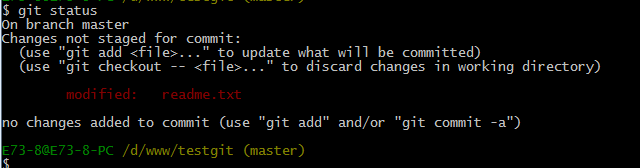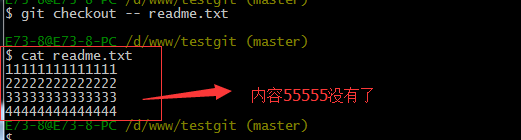### 详解用TensorFlow实现逻辑回归算法，手把手教你使用Git

Git使用教程

``````# Logistic Regression
# 逻辑回归
#----------------------------------
#
# This function shows how to use TensorFlow to
# solve logistic regression.
# y = sigmoid(Ax + b)
#
# We will use the low birth weight data, specifically:
# y = 0 or 1 = low birth weight
# x = demographic and medical history data

import matplotlib.pyplot as plt
import numpy as np
import tensorflow as tf
import requests
from tensorflow.python.framework import ops
import os.path
import csv

ops.reset_default_graph()

# Create graph
sess = tf.Session()

###
# Obtain and prepare data for modeling
###

# name of data file
birth_weight_file = 'birth_weight.csv'

# download data and create data file if file does not exist in current directory
if not os.path.exists(birth_weight_file):
birthdata_url = 'https://github.com/nfmcclure/tensorflow_cookbook/raw/master/01_Introduction/07_Working_with_Data_Sources/birthweight_data/birthweight.dat'
birth_file = requests.get(birthdata_url)
birth_data = birth_file.text.split('\r\n')
birth_data = [[float(x) for x in y.split('\t') if len(x)>=1] for y in birth_data[1:] if len(y)>=1]
with open(birth_weight_file, "w") as f:
writer = csv.writer(f)
writer.writerows(birth_data)
f.close()

# read birth weight data into memory
birth_data = []
with open(birth_weight_file, newline='') as csvfile:
birth_data.append(row)

birth_data = [[float(x) for x in row] for row in birth_data]

# Pull out target variable
y_vals = np.array([x for x in birth_data])
# Pull out predictor variables (not id, not target, and not birthweight)
x_vals = np.array([x[1:8] for x in birth_data])

# set for reproducible results
seed = 99
np.random.seed(seed)
tf.set_random_seed(seed)

# Split data into train/test = 80%/20%
# 分割数据集为测试集和训练集
train_indices = np.random.choice(len(x_vals), round(len(x_vals)*0.8), replace=False)
test_indices = np.array(list(set(range(len(x_vals))) - set(train_indices)))
x_vals_train = x_vals[train_indices]
x_vals_test = x_vals[test_indices]
y_vals_train = y_vals[train_indices]
y_vals_test = y_vals[test_indices]

# Normalize by column (min-max norm)
# 将所有特征缩放到0和1区间（min-max缩放），逻辑回归收敛的效果更好
# 归一化特征
def normalize_cols(m):
col_max = m.max(axis=0)
col_min = m.min(axis=0)
return (m-col_min) / (col_max - col_min)

x_vals_train = np.nan_to_num(normalize_cols(x_vals_train))
x_vals_test = np.nan_to_num(normalize_cols(x_vals_test))

###
# Define Tensorflow computational graph¶
###

# Declare batch size
batch_size = 25

# Initialize placeholders
x_data = tf.placeholder(shape=[None, 7], dtype=tf.float32)
y_target = tf.placeholder(shape=[None, 1], dtype=tf.float32)

# Create variables for linear regression
A = tf.Variable(tf.random_normal(shape=[7,1]))
b = tf.Variable(tf.random_normal(shape=[1,1]))

# Declare model operations

# Declare loss function (Cross Entropy loss)
loss = tf.reduce_mean(tf.nn.sigmoid_cross_entropy_with_logits(logits=model_output, labels=y_target))

# Declare optimizer
train_step = my_opt.minimize(loss)

###
# Train model
###

# Initialize variables
init = tf.global_variables_initializer()
sess.run(init)

# Actual Prediction
# 除记录损失函数外，也需要记录分类器在训练集和测试集上的准确度。
# 所以创建一个返回准确度的预测函数
prediction = tf.round(tf.sigmoid(model_output))
predictions_correct = tf.cast(tf.equal(prediction, y_target), tf.float32)
accuracy = tf.reduce_mean(predictions_correct)

# Training loop
# 开始遍历迭代训练，记录损失值和准确度
loss_vec = []
train_acc = []
test_acc = []
for i in range(1500):
rand_index = np.random.choice(len(x_vals_train), size=batch_size)
rand_x = x_vals_train[rand_index]
rand_y = np.transpose([y_vals_train[rand_index]])
sess.run(train_step, feed_dict={x_data: rand_x, y_target: rand_y})

temp_loss = sess.run(loss, feed_dict={x_data: rand_x, y_target: rand_y})
loss_vec.append(temp_loss)
temp_acc_train = sess.run(accuracy, feed_dict={x_data: x_vals_train, y_target: np.transpose([y_vals_train])})
train_acc.append(temp_acc_train)
temp_acc_test = sess.run(accuracy, feed_dict={x_data: x_vals_test, y_target: np.transpose([y_vals_test])})
test_acc.append(temp_acc_test)
if (i+1)%300==0:
print('Loss = ' + str(temp_loss))

###
# Display model performance
###

# 绘制损失和准确度
plt.plot(loss_vec, 'k-')
plt.title('Cross Entropy Loss per Generation')
plt.xlabel('Generation')
plt.ylabel('Cross Entropy Loss')
plt.show()

# Plot train and test accuracy
plt.plot(train_acc, 'k-', label='Train Set Accuracy')
plt.plot(test_acc, 'r--', label='Test Set Accuracy')
plt.title('Train and Test Accuracy')
plt.xlabel('Generation')
plt.ylabel('Accuracy')
plt.legend(loc='lower right')
plt.show()
``````

Git是目前世界上最先进的分布式版本控制系统。

1.9.2系统提供的log和go-log，产生了对log4go的日志输出进行优化的想法。

Loss = 0.845124
Loss = 0.658061
Loss = 0.471852
Loss = 0.643469
Loss = 0.672077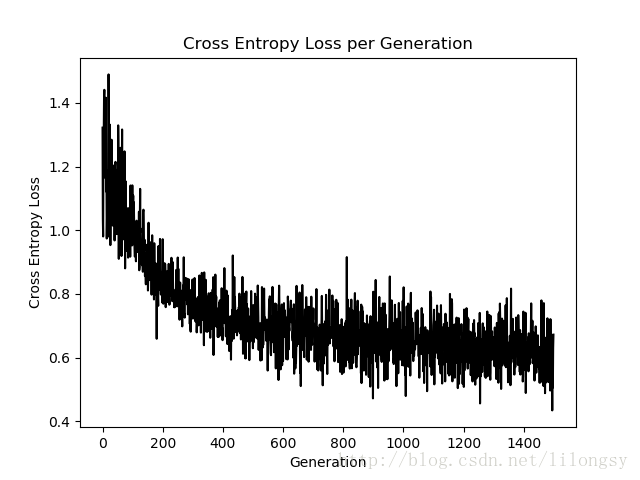SVN是集中式版本控制系统，版本库是集中放在中央服务器的，而干活的时候，用的都是自己的电脑，所以首先要从中央服务器哪里得到最新的版本，然后干活，干完后，需要把自己做完的活推送到中央服务器。集中式版本控制系统是必须联网才能工作，如果在局域网还可以，带宽够大，速度够快，如果在互联网下，如果网速慢的话，就纳闷了。

log使用multiwriter支持多个日志输出，用 Mutex

LogRecord 日志记录。

Git是分布式版本控制系统，那么它就没有中央服务器的，每个人的电脑就是一个完整的版本库，这样，工作的时候就不需要联网了，因为版本都是在自己的电脑上。既然每个人的电脑都有一个完整的版本库，那多个人如何协作呢？比如说自己在电脑上改了文件A，其他人也在电脑上改了文件A，这时，你们两之间只需把各自的修改推送给对方，就可以互相看到对方的修改了。log4go 将屏幕日志输出 termlog

`Close()`时加延时，等待 goroutine 启动来解决。然后还要检查 channel ……

msysgit是 windows版的Git,如下：

``````func (f *Filter) Close() {
if f.closed {
return
}
// sleep at most one second and let go routine running
// drain the log channel before closing
for i := 10; i > 0; i-- {
// Must call Sleep here, otherwise, may panic send on closed channel
time.Sleep(100 * time.Millisecond)
if len(f.rec) <= 0 {
break
}
}
// block write channel
f.closed = true
defer f.LogWriter.Close()
close(f.rec)
if len(f.rec) <= 0 {
return
}
// drain the log channel and write driect
for rec := range f.rec {
f.LogWrite(rec)
}
}
``````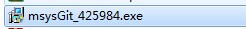log直接将格式化日志信息输出到屏幕，简单多了。

#### 您可能感兴趣的文章:

• 用TensorFlow实现lasso回归和岭回归算法的示例
• TensorFlow实现Softmax回归模型
• 运用TensorFlow进行简单实现线性回归、梯度下降示例
• 用tensorflow构建线性回归模型的示例代码
• 用tensorflow实现弹性网络回归算法
• 用TensorFlow实现戴明回归算法的示例

“Git –> Git Bash”,如下：

writer，直接输出到屏幕。拟将FileLog，SocketLog作为backend，仍然放在结构里。这样，调试小程序和生产程序可以使用同一个日志库。实测效率略有降低。不知道
windows 下的 ColorLog 如何，以后再说。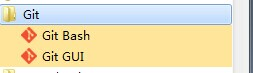Determine caller func – it’s
expensive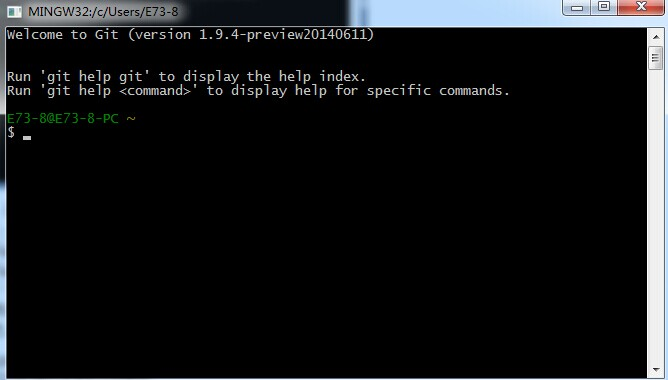。如果要对log4go进行封装，设置`log.SetSkip(log.GetSkip()+1)`

format优化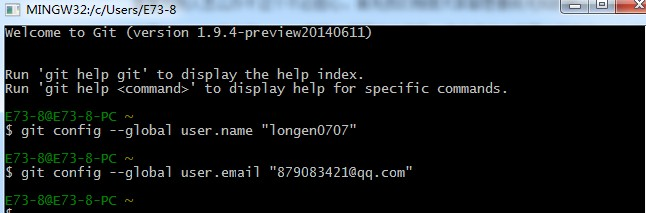• 有一个1秒更新一次的cache机制。很漂亮。
• 大量使用字符串格式化函数——fmt.Sprintf。
• 返回字符串。而writer一般支持的是[]byte。多做一次转换。
• 每次都bytes.Splite讲format字符串以%字符分解成[][]byte。

``````// Cheap integer to fixed-width decimal ASCII. Give a negative width to avoid zero-padding.
func itoa(buf *[]byte, i int, wid int) {
// Assemble decimal in reverse order.
var b byte
bp := len(b) - 1
for i >= 10 || wid > 1 {
wid--
q := i / 10
b[bp] = byte('0' + i - q*10)
bp--
i = q
}
// i < 10
b[bp] = byte('0' + i)
*buf = append(*buf, b[bp:]...)
}
``````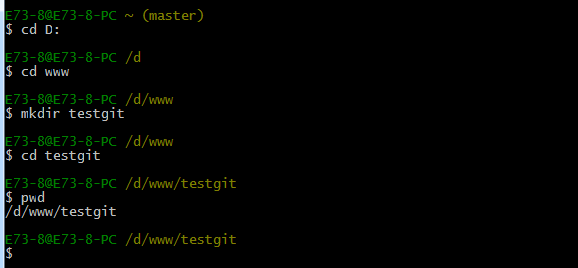``````BenchmarkFormatLogRecord-4    300000    4480 ns/op
BenchmarkConsoleLog-4     1000000    1748 ns/op
BenchmarkConsoleNotLogged-4    20000000    97.5 ns/op
BenchmarkConsoleUtilLog-4     300000    3496 ns/op
BenchmarkConsoleUtilNotLog-4   20000000    104 ns/op
``````

pwd 命令是用于显示当前的目录。

1. 通过命令 git init 把这个目录变成git可以管理的仓库，如下：
``````BenchmarkFormatLogRecord-4  1000000    1443 ns/op
BenchmarkConsoleLog-4   2000000    982 ns/op
BenchmarkConsoleUtilLog-4   500000    3242 ns/op
BenchmarkConsoleUtilNotLog-4 30000000    48.4 ns/op
``````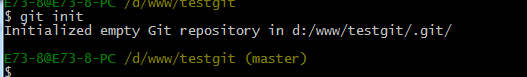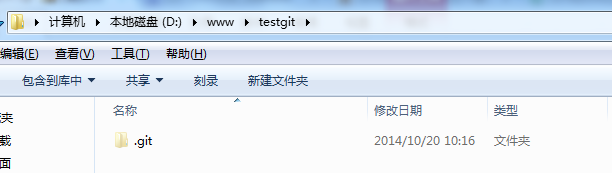BenchmarkConsoleUtilLog调用了`runtime.Caller`，格式化信息，且新增了输出信息到屏幕的时间。

1. 把文件添加到版本库中。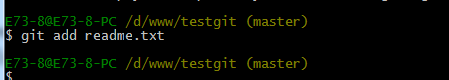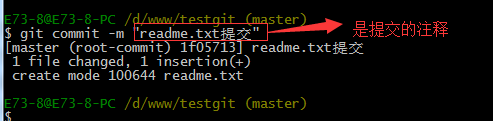status来查看是否还有文件未提交，如下：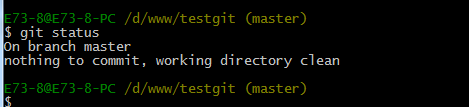status来查看下结果，如下：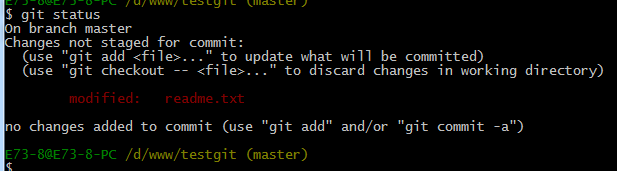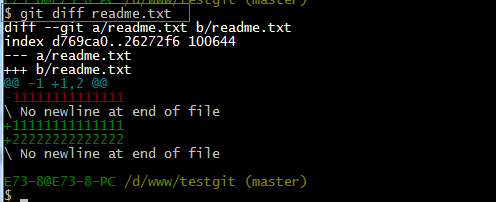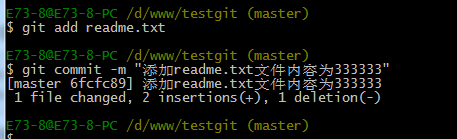git log 演示如下所示：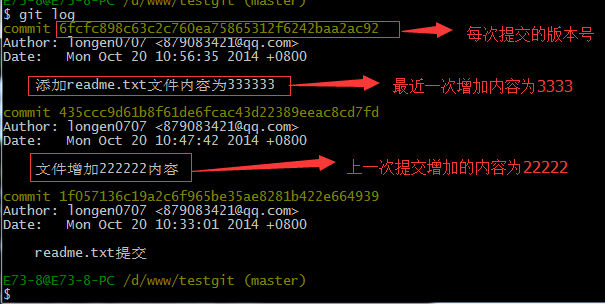git
log命令显示从最近到最远的显示日志，我们可以看到最近三次提交，最近的一次是,增加内容为333333.上一次是添加内容222222，第一次默认是
111111.如果嫌上面显示的信息太多的话，我们可以使用命令 git log
–pretty=oneline 演示如下：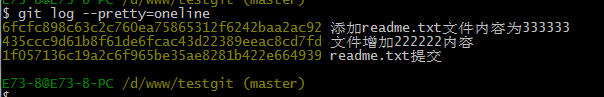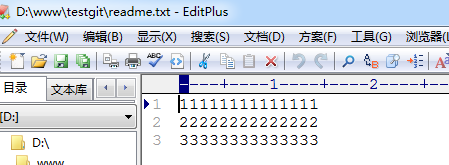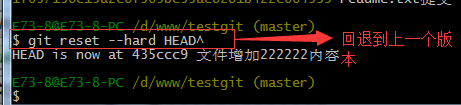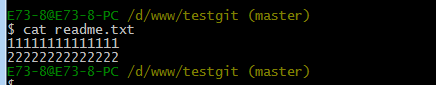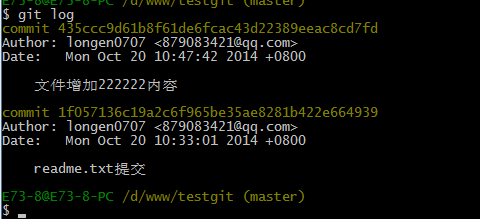git reset –hard 版本号
，但是现在的问题假如我已经关掉过一次命令行或者333内容的版本号我并不知道呢？要如何知道增加3333内容的版本号呢？可以通过如下命令即可获取到版本号：git
reflog 演示如下：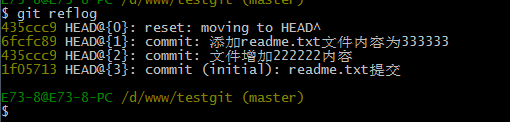6fcfc89.我们现在可以命令

git reset –hard 6fcfc89来恢复了。演示如下：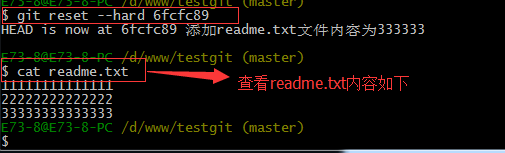commit提交更改，实际上就是把暂存区的所有内容提交到当前分支上。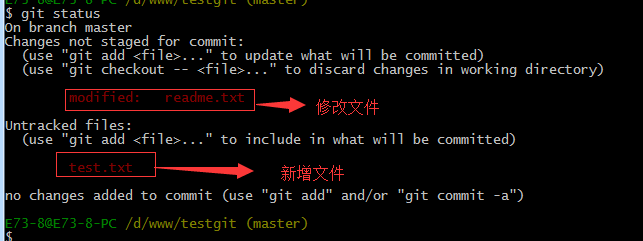status来查看下状态，如下：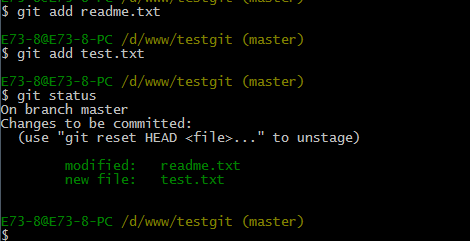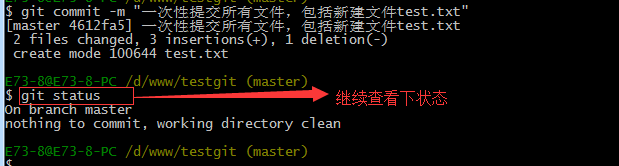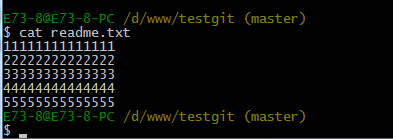git status 查看下当前的状态。如下所示：